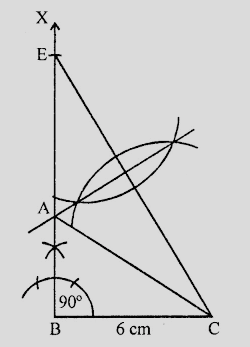# Construct a right triangle $ABC$ whose base $BC$ is $6\ cm$ and the sum of hypotenuse $AC$ and other side $AB$ is $10\ cm$.

Given:

A right triangle $ABC$ whose base $BC$ is $6\ cm$ and the sum of hypotenuse $AC$ and other side $AB$ is $10\ cm$.

To do:

We have to construct the given triangle.

Solution:Steps of construction:

(i) Draw a line segment $BC = 6\ cm$

(ii) At $B$, draw a ray $BX$ making an angle of $90^o$ and cut off $BE = 10\ cm$.

(iii) Join $EC$ and draw the perpendicular bisector of $CE$ which intersects $BE$ at $A$.

(iv) Join $AC$.

Therefore,

$\triangle ABC$ is the required triangle.

Updated on: 10-Oct-2022

31 Views